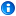### learning goals

The friction force between two surfaces is proportional to the weight.
This is because when the molecules are pressed together more, they interact more and create more resistance.
If the surfaces are rough, they lock together more and it's harder for them to slide past each other.
When you move an object, the force to push it is the same as the force to pull it.

#### Discovery question

How strong is the force of friction?

Cannot resolve external resource into attachment.
Measure the amount of force needed to make a box start to move and continue to move.

#### Engagetie-in to story. focus on microscopic surface qualities

He decided his experiment would look like this:

Cannot resolve external resource into attachment.

Here is a graph of force against time. Suppose you pushed very lightly on the force sensor, then harder and harder until the box started sliding. Predict what your graph would look like.
PREDICTION GRAPH

Suppose the box was twice as heavy. How would the graph be different?

#### Materials

• Force sensor
• Cardboard box just large enough to hold three water bottles
• Three water bottles
• Sandpaper

#### Explore

Find a small cardboard box. Find water bottles such that three can fit into the box. Fill them with water. Put one bottle into the box.

Attach the force sensor to the computer.

Cannot resolve external resource into attachment.

Start recording with the force sensor. Push the box with the force sensor. Gradually push harder and harder until the box starts to slide. Keep pushing so that the box slides at a constant speed.
FORCE SENSOR GRAPH with prediction, multiple datasets: prediction / one / two / three

Stop recording. Describe your graph. What did it look like before the box started moving? What happened just when the box started moving? What happened after that?

Label your graph. Mark where you first started pushing. Mark where the box first started to move. Mark the greatest force value.
GRAPH LABELING technical hints

What was the greatest force value and when did it happen?

Record the average value of the moving friction force.

TABLE
bottles Friction force
one
two
three

Now put two water bottles in the box.
Cannot resolve external resource into attachment.

Select dataset "two" and repeat your experiment. Be sure to label the new line you have made on your graph.
FORCE SENSOR GRAPH - repeat of above, multiple datasets

Record the average value of the moving friction force.

TABLE (repeat from above)
bottles Friction force
one
two
three

#### Explain

Why do you think the friction force increased when the weight increased?

TABLE (repeat from above)
bottles Friction force
one
two
three

Is the friction force with two bottles about twice the friction force with one bottle?

• yes
• no

How can you tell?

(scaffold: want them to explain their math, such as, when I multiply the first number by two, it is close to (or not close to) the second number. Is this too elementary?)

Divide the larger by the smaller. What is the exact ratio?
force with two bottles / force with one bottle =

#### Elaborate

TABLE (repeat from above)
bottles Friction force
one
two
three

Use the values you collected to predict what the friction force would be with three bottles of water in the box. The friction force with three bottles would be:

Now put three water bottles in the box. Select dataset "three" and repeat your experiment. Be sure to label the new line you have made on your graph.
FORCE SENSOR GRAPH - repeat of above, multiple datasets

Record the average value of the moving friction force.

TABLE (repeat from above)
bottles Friction force
one
two
three

Is the friction force for three bottles about three times the friction force for one bottle?
yes
no

Calculate the exact ratio.
force with three bottles / force with one bottle =

#### Evaluate

Suppose you could zoom in to where the box surface touches the table top. Draw what you think the two surfaces would look like.
DRAW TOOL

What causes the friction between the two surfaces?Document generated by Confluence on Jan 27, 2014 16:49